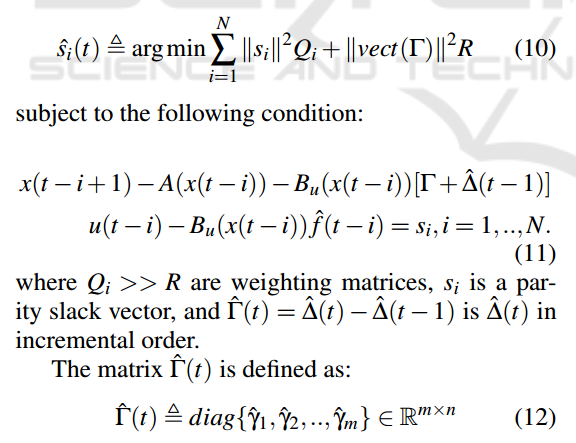# How to define the following cost function in correct DCP format?

Hi all,
I have a following functionto solve in cvxpy. While setting the objective functions I am getting the DCP rule voilation error. My script is below.

``````deltaButOld=np.array([1,1,1,1]).T
deltabut1=cp.Variable()
deltabut2=cp.Variable()
deltabut3=cp.Variable()
deltabut4=cp.Variable()
deltaBut=np.array([deltabut1,deltabut2,deltabut3,deltabut4]).T

objective1=0
s_i=np.eye(3)
s1=cp.Variable((3,N))       #N=5

for i in range (0,N):

objective2=(deltaBut-deltaButOld)
d_i=np.eye(4)

objective=objective1+objective2_final
problem=cp.Problem(cp.Minimize(objective))
problem.solve()
``````

from the solution, I need to get the optimal values of deltabut1…deltabut4.
Could anybody help me to define the above cost function in proper DCP format??
Thank you very much in advance!

1 Like

CVXPY is a different tool than CVX. I think you are now encouraged to post CVXPY help requests at https://discord.com/invite/SWUSgBu3Yz .

Since your objective/cost function is a minimum , can you confirm or proof ,this is equates to a convex function and thereby recode your program using cvx . In addition can you showcase your paper for better understanding over your optimization problem .